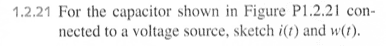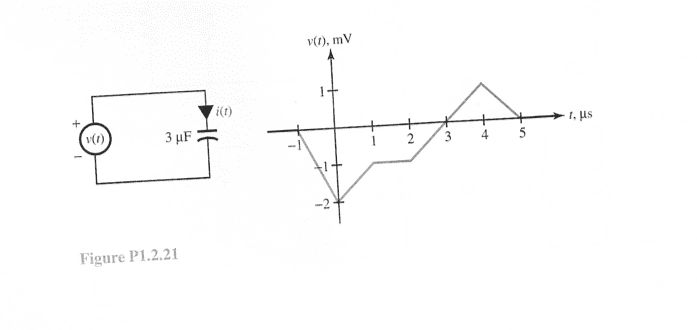# Circuits: Current and Energy of Capacitor

## Homework Statement

I am just looking for someone to verify that my approach is correct.
i(t) is the current through the capacitor and w(t) is the energy stored in the capacitor.## Homework Equations

For a capacitor, the current through it can be related to the voltage drop across the capacitor:

$$i_c = C\frac{dv_c}{dt}$$

where C is the capacitance (a constant in this case).

## The Attempt at a Solution

The source voltage is given (as function of time) pictorially in figure 1.2.21. From Kirchoff's voltage law, we know that $v_c(t) = -v_{source}(t)$ Hence we can find the slope $dv_c/dt = -dv_{source}/dt$.

From the figure, from t = -1 to t =0, the slope of the source voltage is 2, hence the capacitor voltage's slope is -2. So the current through the capacitor is -2*C from t = -1 to 0.

Homework Helper
I agree with that answer and approach.
It does seem that there are two extra minus signs in there, though. The voltage on the capacitor is the same as the voltage on the generator - no negative. In fact, when you put your voltmeter across the generator, you are also putting it across the capacitor. I guess there would be a negative sign necessary if you are switching the leads when you measure the voltage on the capacitor.

The slope on the graph is negative 2, not positive 2.

I agree with that answer and approach.
It does seem that there are two extra minus signs in there, though. The voltage on the capacitor is the same as the voltage on the generator - no negative. In fact, when you put your voltmeter across the generator, you are also putting it across the capacitor. I guess there would be a negative sign necessary if you are switching the leads when you measure the voltage on the capacitor.

The slope on the graph is negative 2, not positive 2.

Hmmm.... The slope on the source voltage graph is -2. That is why I think that the slope of the voltage of the capacitor is positive 2.

KVL implies that vsource + vcap = 0 yes?

Homework Helper
We are looking at things slightly differently, both correct!
I would naturally put the red lead of the voltmeter on the top of the capacitor and the black on the bottom. Same for the source. You are going around the circuit in a clockwise direction, keeping the black lead ahead of the red, as you would when using the rule that the sum of the voltages around a closed loop is zero.

Anyway, the top of the capacitor is positive and the (positive) current flows down through it.